# 2017-12, Keio University, Projection-based Regularized Dual Averaging for Stochastic Optimization with Its Applications to Classification and Regression

Dec. 24, 2021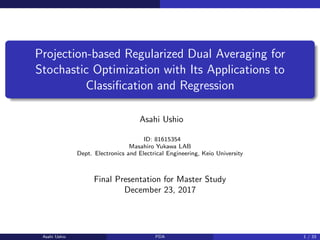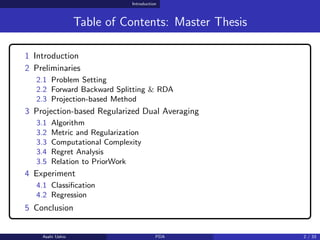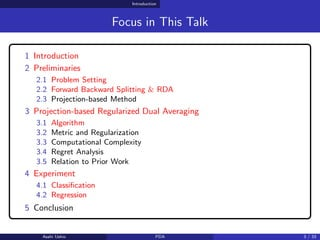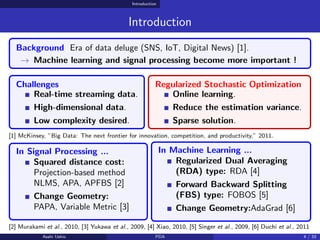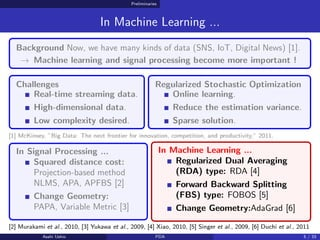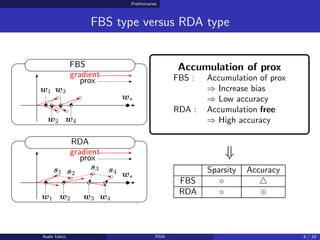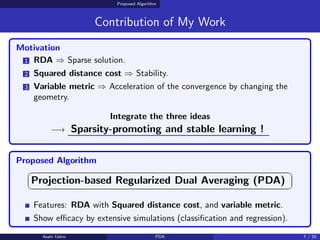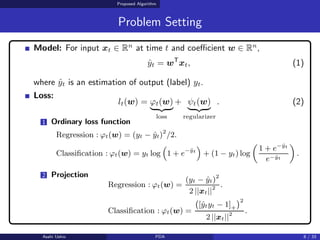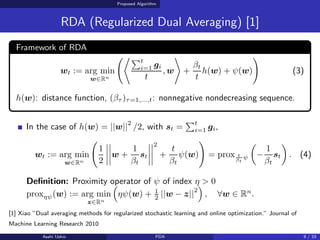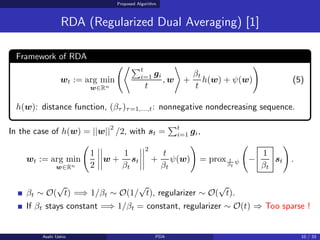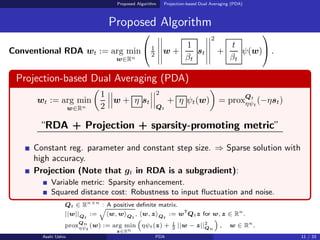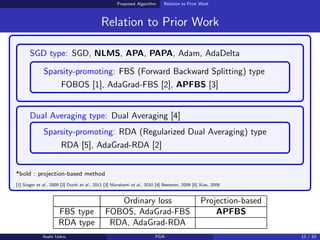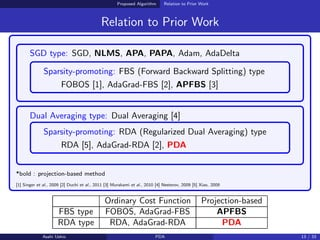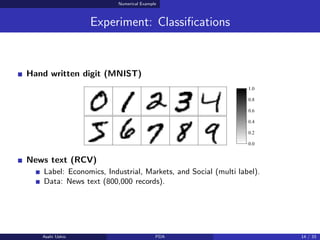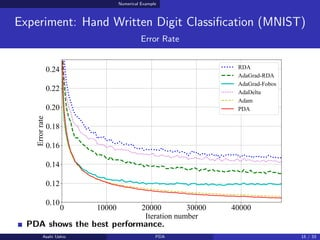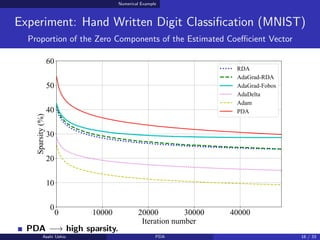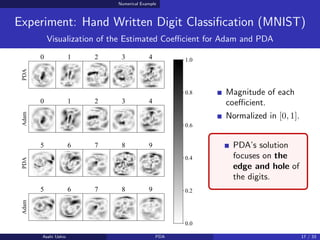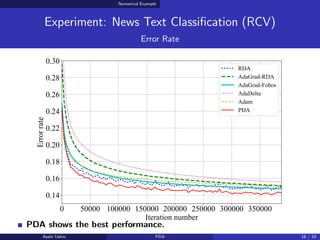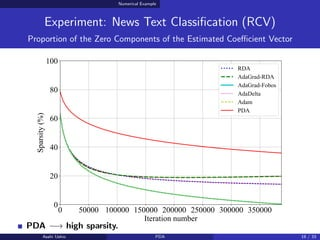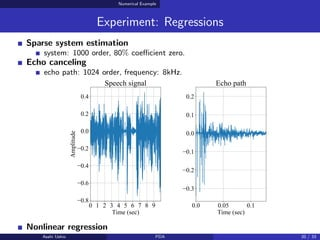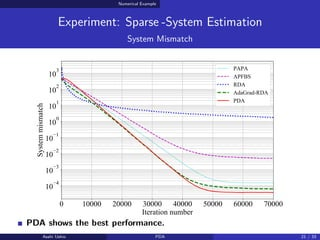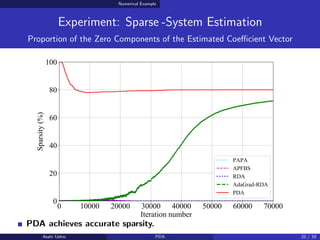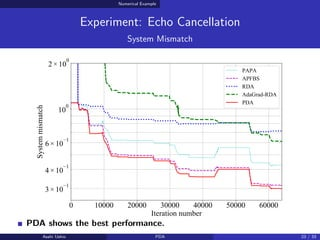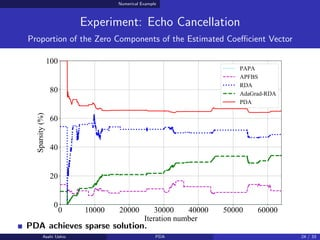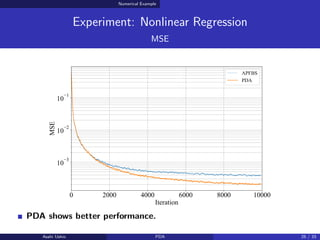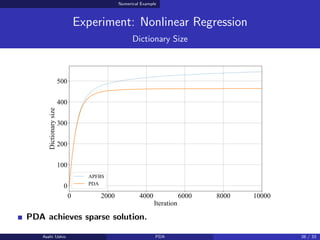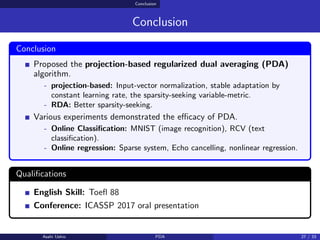1 of 27

### 2017-12, Keio University, Projection-based Regularized Dual Averaging for Stochastic Optimization with Its Applications to Classification and Regression

• 1. Projection-based Regularized Dual Averaging for Stochastic Optimization with Its Applications to Classification and Regression Asahi Ushio ID: 81615354 Masahiro Yukawa LAB Dept. Electronics and Electrical Engineering, Keio University Final Presentation for Master Study December 23, 2017 Asahi Ushio PDA 1 / 33
• 2. Introduction Table of Contents: Master Thesis 1 Introduction 2 Preliminaries 2.1 Problem Setting 2.2 Forward Backward Splitting & RDA 2.3 Projection-based Method 3 Projection-based Regularized Dual Averaging 3.1 Algorithm 3.2 Metric and Regularization 3.3 Computational Complexity 3.4 Regret Analysis 3.5 Relation to PriorWork 4 Experiment 4.1 Classification 4.2 Regression 5 Conclusion Asahi Ushio PDA 2 / 33
• 3. Introduction Focus in This Talk 1 Introduction 2 Preliminaries 2.1 Problem Setting 2.2 Forward Backward Splitting & RDA 2.3 Projection-based Method 3 Projection-based Regularized Dual Averaging 3.1 Algorithm 3.2 Metric and Regularization 3.3 Computational Complexity 3.4 Regret Analysis 3.5 Relation to Prior Work 4 Experiment 4.1 Classification 4.2 Regression 5 Conclusion Asahi Ushio PDA 3 / 33
• 4. Introduction Introduction Background Era of data deluge (SNS, IoT, Digital News) . → Machine learning and signal processing become more important ! Challenges Real-time streaming data. High-dimensional data. Low complexity desired. Regularized Stochastic Optimization Online learning. Reduce the estimation variance. Sparse solution.  McKinsey, ”Big Data: The next frontier for innovation, competition, and productivity,” 2011. In Signal Processing ... Squared distance cost: Projection-based method NLMS, APA, APFBS  Change Geometry: PAPA, Variable Metric  In Machine Learning ... Regularized Dual Averaging (RDA) type: RDA  Forward Backward Splitting (FBS) type: FOBOS  Change Geometry:AdaGrad   Murakami et al., 2010,  Yukawa et al., 2009,  Xiao, 2010,  Singer et al., 2009,  Duchi et al., 2011 Asahi Ushio PDA 4 / 33
• 5. Preliminaries In Machine Learning ... Background Now, we have many kinds of data (SNS, IoT, Digital News) . → Machine learning and signal processing become more important ! Challenges Real-time streaming data. High-dimensional data. Low complexity desired. Regularized Stochastic Optimization Online learning. Reduce the estimation variance. Sparse solution.  McKinsey, ”Big Data: The next frontier for innovation, competition, and productivity,” 2011. In Signal Processing ... Squared distance cost: Projection-based method NLMS, APA, APFBS  Change Geometry: PAPA, Variable Metric  In Machine Learning ... Regularized Dual Averaging (RDA) type: RDA  Forward Backward Splitting (FBS) type: FOBOS  Change Geometry:AdaGrad   Murakami et al., 2010,  Yukawa et al., 2009,  Xiao, 2010,  Singer et al., 2009,  Duchi et al., 2011 Asahi Ushio PDA 5 / 33
• 6. Preliminaries FBS type versus RDA type grad grad FBS RDA gradient gradient prox prox w∗ w∗ w1 w1 w2 w2 w3 w3 w4 w4 s1 s2 s3 s4 Accumulation of prox FBS : Accumulation of prox ⇒ Increase bias ⇒ Low accuracy RDA : Accumulation free ⇒ High accuracy ⇓ Sparsity Accuracy FBS ◦ △ RDA ◦ ! Asahi Ushio PDA 6 / 33
• 7. Proposed Algorithm Contribution of My Work Motivation 1 RDA ⇒ Sparse solution. 2 Squared distance cost ⇒ Stability. 3 Variable metric ⇒ Acceleration of the convergence by changing the geometry. Integrate the three ideas −→ Sparsity-promoting and stable learning ! Proposed Algorithm ✞ ✝ ☎ ✆ Projection-based Regularized Dual Averaging (PDA) Features: RDA with Squared distance cost, and variable metric. Show eﬃcacy by extensive simulations (classification and regression). Asahi Ushio PDA 7 / 33
• 8. Proposed Algorithm Problem Setting Model: For input xt ∈ Rn at time t and coeﬃcient w ∈ Rn , ŷt = wT xt, (1) where ŷt is an estimation of output (label) yt. Loss: lt(w) = ϕt(w) ! "# \$ loss + ψt(w) ! "# \$ regularizer . (2) 1 Ordinary loss function Regression : ϕt(w) = (yt − ŷt)2 /2. Classification : ϕt(w) = yt log ! 1 + e−ŷt " + (1 − yt) log # 1 + e−ŷt e−ŷt \$ . 2 Projection Regression : ϕt(w) = (yt − ŷt)2 2 ||xt||2 . Classification : ϕt(w) = % [ŷtyt − 1]+ &2 2 ||xt||2 . Asahi Ushio PDA 8 / 33
• 9. Proposed Algorithm RDA (Regularized Dual Averaging)  Framework of RDA wt := arg min w∈Rn %&'t i=1 gi t , w ( + βt t h(w) + ψ(w) ) (3) h(w): distance function, (βτ )τ=1,...,t: nonnegative nondecreasing sequence. In the case of h(w) = ||w||2 /2, with st = 't i=1 gi, wt := arg min w∈Rn % 1 2 * * * * * * * *w + 1 βt st * * * * * * * * 2 + t βt ψ(w) ) = prox t βt ψ + − 1 βt st , . (4) Definition: Proximity operator of ψ of index η > 0 proxηψ(w) := arg min z∈Rn - ηψ(w) + 1 2 ||w − z||2 . , ∀w ∈ Rn .  Xiao ”Dual averaging methods for regularized stochastic learning and online optimization.” Journal of Machine Learning Research 2010 Asahi Ushio PDA 9 / 33
• 10. Proposed Algorithm RDA (Regularized Dual Averaging)  Framework of RDA wt := arg min w∈Rn %&'t i=1 gi t , w ( + βt t h(w) + ψ(w) ) (5) h(w): distance function, (βτ )τ=1,...,t: nonnegative nondecreasing sequence. In the case of h(w) = ||w||2 /2, with st = 't i=1 gi, wt := arg min w∈Rn % 1 2 * * * * * * * *w + 1 βt st * * * * * * * * 2 + t βt ψ(w) ) = prox t βt ψ % − 1 βt st ) . βt ∼ O( √ t) =⇒ 1/βt ∼ O(1/ √ t), regularizer ∼ O( √ t). If βt stays constant =⇒ 1/βt = constant, regularizer ∼ O(t) ⇒ Too sparse ! Asahi Ushio PDA 10 / 33
• 11. Proposed Algorithm Projection-based Dual Averaging (PDA) Proposed Algorithm Conventional RDA wt := arg min w∈Rn ⎛ ⎝1 2 * * * * * * * * * * w + 1 βt st * * * * * * * * * * 2 + t βt ψ(w) ⎞ ⎠ . Projection-based Dual Averaging (PDA) wt := arg min w∈Rn + 1 2 * * * * * *w + η st * * * * * * 2 Qt + η ψt(w) , = proxQt ηψt (−ηst) “RDA + Projection + sparsity-promoting metric” Constant reg. parameter and constant step size. ⇒ Sparse solution with high accuracy. Projection (Note that gt in RDA is a subgradient): Variable metric: Sparsity enhancement. Squared distance cost: Robustness to input fluctuation and noise. Qt ∈ Rn×n : A positive definite matrix. ||w||Qt := ! ⟨w, w⟩Qt , ⟨w, z⟩Qt := wT Qtz for w, z ∈ Rn . proxQn ηψt (w) := arg min z∈Rn " ηψt(z) + 1 2 ||w − z||2 Qn # , w ∈ Rn . Asahi Ushio PDA 11 / 33
• 14. Numerical Example Experiment: Classifications Hand written digit (MNIST) News text (RCV) Label: Economics, Industrial, Markets, and Social (multi label). Data: News text (800,000 records). Asahi Ushio PDA 14 / 33
• 15. Numerical Example Experiment: Hand Written Digit Classification (MNIST) Error Rate PDA shows the best performance. Asahi Ushio PDA 15 / 33 proposed
• 16. Numerical Example Experiment: Hand Written Digit Classification (MNIST) Proportion of the Zero Components of the Estimated Coeﬃcient Vector PDA −→ high sparsity. Asahi Ushio PDA 16 / 33 proposed
• 17. Numerical Example Experiment: Hand Written Digit Classification (MNIST) Visualization of the Estimated Coeﬃcient for Adam and PDA Magnitude of each coeﬃcient. Normalized in [0, 1]. PDA’s solution focuses on the edge and hole of the digits. Asahi Ushio PDA 17 / 33
• 18. Numerical Example Experiment: News Text Classification (RCV) Error Rate PDA shows the best performance. Asahi Ushio PDA 18 / 33 proposed
• 19. Numerical Example Experiment: News Text Classification (RCV) Proportion of the Zero Components of the Estimated Coeﬃcient Vector PDA −→ high sparsity. Asahi Ushio PDA 19 / 33 proposed
• 20. Numerical Example Experiment: Regressions Sparse system estimation system: 1000 order, 80% coeﬃcient zero. Echo canceling echo path: 1024 order, frequency: 8kHz. Nonlinear regression Asahi Ushio PDA 20 / 33
• 21. Numerical Example Experiment: Sparse -System Estimation System Mismatch PDA shows the best performance. Asahi Ushio PDA 21 / 33 proposed
• 22. Numerical Example Experiment: Sparse -System Estimation Proportion of the Zero Components of the Estimated Coeﬃcient Vector PDA achieves accurate sparsity. Asahi Ushio PDA 22 / 33 proposed
• 23. Numerical Example Experiment: Echo Cancellation System Mismatch PDA shows the best performance. Asahi Ushio PDA 23 / 33 proposed
• 24. Numerical Example Experiment: Echo Cancellation Proportion of the Zero Components of the Estimated Coeﬃcient Vector PDA achieves sparse solution. Asahi Ushio PDA 24 / 33 proposed
• 25. Numerical Example Experiment: Nonlinear Regression MSE PDA shows better performance. Asahi Ushio PDA 25 / 33 proposed
• 26. Numerical Example Experiment: Nonlinear Regression Dictionary Size PDA achieves sparse solution. Asahi Ushio PDA 26 / 33 proposed
• 27. Conclusion Conclusion Conclusion Proposed the projection-based regularized dual averaging (PDA) algorithm. - projection-based: Input-vector normalization, stable adaptation by constant learning rate, the sparsity-seeking variable-metric. - RDA: Better sparsity-seeking. Various experiments demonstrated the eﬃcacy of PDA. - Online Classification: MNIST (image recognition), RCV (text classification). - Online regression: Sparse system, Echo cancelling, nonlinear regression. Qualifications English Skill: Toefl 88 Conference: ICASSP 2017 oral presentation Asahi Ushio PDA 27 / 33